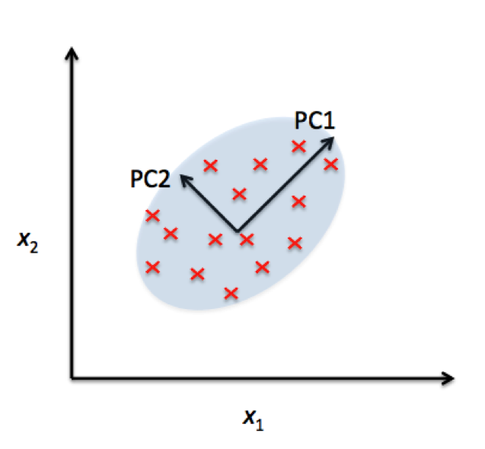/  Top Machine learning interview questions and answers   /  What is the difference between Multi-Dimensional Scaling and Principal Component Analysis?## What is the difference between Multi-Dimensional Scaling and Principal Component Analysis?

Principal Component Analysis

The input to PCA is the original vectors in n-dimensional space.

And the data are projected onto the directions in the data with the most variance. Hence the “spread” of the data is roughly conserved as the dimensionality decreases.

Multidimensional Scaling

The input to MDS is the pairwise distances between points.

The output of MDS is a two- or three-dimensional projection of the points where distances are preserved.